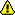You should trust both wikis because the password could be read by the particular administrators.

Clear message

# Importing 3D Maps from the PDB

There has arisen an issue concerning importing from pdb, transformation to the Brix format, and the rotations that chimera performs relative to this format. We will describe the relationships between the transformations applied within SPARX to the transformations performed in Chimera.

Facts to help sort out the motivation of Phil's struggle on the transformation When dealing with fitting crystal piece into HRS density map:

• 1. Convert a PDB file into SPIDER density map, load it in Chimera;
• convert the SPIDER formatted structure into BRIX format, also load it in Chimera; load PDB file into Chimera, and compare them: you will find the orientation of the structure in SPDIER format is
• different from the one of structure in BRIX format; while the orientation of the structure
• in BRIX format is identical to the one in PDB format.
• 2. We speculate there is a hidden transformation when loading in SPIDER format structure in Chimera:
• `R_alpha`. So, to rotate SPDIER formated structure, `R_gamma`, and load it in chimera; `R_alpha*R_gamma` We can also convert it into BRIX format, and load it in Chimera, and rotate it ;

• `R_sigma*R_alpha` The rotations should be equivalent (have the same displayment), so `R_sigma*R_alpha = R_alpha*R_gamma ` `R_sigma = R_alpha *R_gamma * R_alpha ^(-1)`

# Capturing the matrices that represent the current state

If, say, a Spider file is opened in Chimera, and the show command from the model panel is used, then Chimera will record the transformations that are performed manually with the mouse using a command such as > matrixget R from the Chimera command line which writes the current transformation, from the point at which the 3D map was opened, to the current state. (The transformation, R, can be reused later in a command such as >matrixset R )

The transformation R is written to a file and can be viewed. The question is

# Solution method (Summer 2005)

To go back and forth from the SPARX (`psi_S,theta_S, phi_S `)convention and what Chimera records( `psi_C, theta_C, phi_C` ):

• `psi_S =  pi/2 - psi_C`

• `theta_S = theta_C`

• `phi_S = (3 pi)/2 - phi_C`

• In more detail about the relation derivation, please check the following notes ( Solution From Summer 2005):
• I created a 3 dimensional tripod with legs with three different lengths.

1. I took the tripod, saved to BRIX, and loaded into Chimera

2. Noting the original positions of the tripods legs,

• I could perform rotations around what were the original definition of the axes.

3. For each of these rotations, a, I noted the transformation recorded in Chimera as b.

• In practice, I used three rotations (positive 90 degrees about each of the original coordinate axes).

4.

• I supposed
• a = `R_alpha` b `R_beta`

for each of the rotations, a, with `R_alpha` and `R_beta` unknown but fixed.

The general solution to these sort of problems is not so easy.

But in this particular case, the problem is not quite so hard and I found (with a certain amount of guesswork):

• `R_beta  = R_alpha = 0 1 0, 1  0  0 , 0  0 -1 `

Notice that `R_beta`is its own inverse `R_beta = R_beta^(-1)` . So a = `R_beta` b `R_beta` and b = `R_beta` a `R_beta`